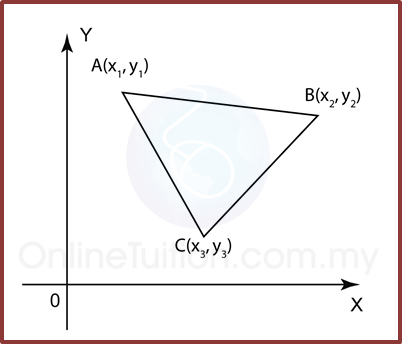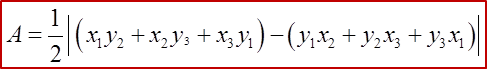# 6.3 Areas of Polygons

6.3 Areas of Polygons

(A) Area of TriangleArea of Triangle1. When the given points are taken in an anticlockwise direction the result is positive; taken in a clockwise direction the result is negative. The answer for the area must be given as a positive value.

Example 1:
Calculate the area of ∆ ABC with the vertices A (-5, 5), B (-2, -4),
C (4, -1).

Solution:

(B) Area of QuadrilateralArea of Quadrilateral(C) If the points A, B and C are collinear, then the area of ∆ ABC is 0.

Example 2:
Find the values of k if the points P (2, 1), Q (6, k) and R (3k, $\frac{9}{2}$ ) are collinear.

Solution: